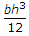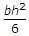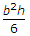# Civil Engineering - Strength of Materials - Discussion

### Discussion :: Strength of Materials - Section 1 (Q.No.1)

1.

A rectangular bar of width b and height h is being used as a cantilever. The loading is in a plane parallel to the side b. The section modulus is

 [A].[B].[C].[D]. none of these.

Explanation:

No answer description available for this question.

 Mukesh Yadav said: (Apr 23, 2013) Section modulus = I/Y(max). Then I = (b^3*h)/12. And y = b/2. Then z = (b^2*h)/6.

 Mahesh said: (May 21, 2013) Section modulus = I/y(max). Here the load is parallel to the width b so, I = (b^3*h)/12. And y = b/2. Then z = (b^2*h)/6.

 C.Mallireddy said: (Jul 16, 2013) Section modulus = I/Y (this is max). Moment of inertia (I) = (d*b^3)/12. = (h*b^3)/12. In this problem width = b. Depth d = h. And y = b/2. :- Z= ( (h*b^3)/12)/(b/2). Z = (h*b^2)/6.

 Prem Kumar Meena said: (Sep 1, 2013) Z = I/Y(max.) I = bd^3/12 ; y(max.) = d/2 load is parallel to side b then b=h and d=b ; Z = hb^3/12/b/2 ; Z = hb^2/6.

 Deepak Singh said: (Oct 11, 2014) We know, Z = I/Y [where, y=y(max)]. But, I = db^3/12. y = b/2. Now, Z = 2db^3/12d, = db^2/6 [A/C to question d=h], Z = hb^2/6.

 Raj said: (Dec 10, 2014) Can somebody explain me "plane parallel to side b"?

 Josh said: (Jan 3, 2015) Its like load is normal to height h which means parallel to b.

 Ithi said: (Jan 30, 2015) (hb^3)% (0.5b) = (hb^2%6).

 Rima said: (Jun 23, 2015) Section modulus = I/Ymax. Here I = h*b^3/12. And Ymax = b/2, then section modulus= (h*b^3/12)/(b/2) = h*b^2/6.

 Gyan Prakash Tiwari said: (Jul 6, 2015) If b is width and h is height then section of modulus. z = I/Y ; I = hb^3/12 and y = b/2 then. z = (hb^3/12)/(b/2). Then z = (b^2/6) is answer. Because the load is in a plane to the side b.

 Ooha said: (Jul 15, 2015) We are using different methods but same concept. So your process is correct no comment.

 Rehan Rufead said: (Jul 22, 2016) Section modulus for the rectangular section is 1/6 bd^2. ie I/y. I = bd^3/12. Y = d/2 bd^2/6. Then, how the answer is option C.

 Devendranaik said: (Sep 2, 2016) Section modules (z) =I/Y. MOMENT OF INERTIA (I) =B^3h/12. Y = b/2. Therefore = Z = I/Y = B^2h/6.

 Aftershock said: (Oct 20, 2016) It says parallel to the plan b, that means. I = bh^3/12. y = b/2, Hence Z= b^2h/6.

 Ghanshyam said: (Nov 20, 2016) Please explain that what is y and how y = b/2? And how calculation is z = {(h*b^3/12)/(b/2).

 Ghanshyam said: (Nov 20, 2016) What is section module?

 Satyendra said: (Jan 7, 2017) Section modulus is a geometric property for a given cross-section used in the design of beams or flexural members.

 Ummar Basha said: (May 16, 2017) Here, bd^3/12. Because I xx of rectangular section.

 Rpr said: (Aug 10, 2017) Z= (Moment of Inertia )/(Depth of NA). = (bh3/12)/(h/2)=bh2/6.

 Samir Sahoo said: (Dec 16, 2017) Thank you for the explanation.

 Priya said: (Jan 2, 2018) Please explain that when section modulus bh2/6?

 Aaron said: (Jan 14, 2018) How y=b/2?

 Riyas said: (Jan 25, 2018) Section modulus is the ratio of MOI of N.A & Distance from NA from the extreme stressed fibre. Here NA plays the main role. The load applied in the face of Length x depth. This face elongated & opposite face compressed. So, NA will lie in between this. That means NA will pass perpendicular to width. so MOI will be b^3.d/12. Distance from n.a. to extreme stressed fibre is b/2.

 Jeldi said: (Jan 29, 2018) In general terms b= width and d or h = depth or height respectively. When loading is applied we consider bd^3/12 (hear loading is parallel to depth) and y=d/2. Hear in this problem, Parallel to width is asked making it shifting terms from width as depth (assume that u have rotated your beam) So hear instead of bd^3 /12 and y=d/2. V get db^3/12 and y= b/2. Making Z=I/Y as db^2/6.

 Star Guy said: (Feb 4, 2018) Thanks for explaining in an uderstandable manner @Riyas.

 James said: (Aug 13, 2018) The correct Answer must be (bd3/12)/(d/2) which means bd2/6.

 Subrata Pal said: (Aug 26, 2018) Thanks for all the given explanation.

 Loki said: (Sep 4, 2018) Loading is parallel to side b then neural axis is perpendicular to side b, I about n.a ( n.a is the axis parallel to bending direction by right-hand thumb rule ) when the plane of loading is symmetry.

 Avi said: (Jan 3, 2019) I think, option B is correct.

 Elias said: (Apr 3, 2019) Thank all.

 Akshay Malviya said: (Jun 20, 2019) Here in the question, it is written that load is in the "plane parallel to side b". Neutral axis should be parallel to b and the correct answer should be option B.

 Pradeep Karsh said: (Aug 30, 2019) Thaks to all.

 Sai Chandu said: (Apr 24, 2020) @Mukesh Yada. Please explain How y=b/2?

 Anantharami Reddy said: (Apr 29, 2020) Option B is correct.

 Jothi said: (May 4, 2020) Section Modulus Z=I/ymax in general I= bd3/12 in this problem d=b ,b=h. The loading is in a plane parallel to the side b. ymax= b/2, Z= hb3/12/b/2 =b2h/6.

 Venkatesh said: (Dec 2, 2020) Option B is the correct answer.

 Poluri Naresh said: (Dec 12, 2020) Options B is the correct answer.